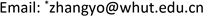MOSModeling and Simulation2324-8696Scientific Research Publishing10.12677/MOS.2021.101016MOS-40679MOS20210100000_46503308.pdf信息通讯 工程技术 水翼尾缘摆动建模与流体动力特性研究 Modeling and Hydrodynamic Characteristics of Flapping Hydrofoil Trailing Edge 21志鸿21忠建21咏鸥2*武汉理工大学，湖北 武汉null030220211001150159© Copyright 2014 by authors and Scientific Research Publishing Inc. 2014This work is licensed under the Creative Commons Attribution International License (CC BY). http://creativecommons.org/licenses/by/4.0/

Modeling and Hydrodynamic Characteristics of Flapping Hydrofoil Trailing Edge<sup> </sup>

Tong Su, Zhihong Wang, Zhongjian Lin, Yongou Zhang*

School of Transportation, Wuhan University of Technology, Wuhan HubeiReceived: Sep. 11th, 2020; accepted: Feb. 17th, 2021; published: Feb. 25th, 2021ABSTRACT

The hydrofoil in the swinging state is widely used in the fields of propeller, wave energy capture and bionic propulsion, and its hydrodynamic characteristic is one of the factors that must be considered in the design of the device. In this paper, the hydrodynamic characteristics of the flapping hydrofoil under different swing angles and different incoming flow speeds are simulated and analyzed. First, the NACA0012 airfoil is established as the analysis object, and then the hydrodynamic calculation method of the flapping hydrofoil is obtained by using the polyhedral grid and the dynamic grid technology. On this basis, the hydrodynamic characteristics of the hydrofoil in the swing angle range of ±20˚ and the flow velocity range of 1~10 m/s are simulated and analyzed. The results show that as the swing angle increases, the maximum pressure changes less, the minimum pressure gradually increases, both lift and resistance increase, the speed of lift increase gradually decreases, and the speed of resistance increase gradually increases. With the increase of the incoming flow velocity, the maximum pressure and the minimum pressure (negative pressure) both increase to varying degrees, the lift and resistance increase, and the increasing speed gradually increases.

Keywords:Hydrofoil, Trailing Edge Swing, Hydrodynamic Characteristics

Copyright © 2021 by author(s) and Hans Publishers Inc.

This work is licensed under the Creative Commons Attribution International License (CC BY 4.0).

http://creativecommons.org/licenses/by/4.0/1. 引言

2. 几何模型2.1. 水翼几何

2.2. 计算工况

Computational case

1±55
2±105
3±205
4±101
5±105
6±1010

3. 计算模型3.1. 控制方程

∂ ρ ∂ t + ∇ ⋅ ( ρ V → ) = 0 (1)

∂ ( ρ u ) ∂ t + ∇ ⋅ ( ρ u V → ) = ∂ p ∂ x + ∂ τ x x ∂ x + ∂ τ y x ∂ y + ∂ τ z x ∂ z + ρ f x (2)

∂ ( ρ v ) ∂ t + ∇ ⋅ ( ρ v V → ) = − ∂ p ∂ y + ∂ τ x y ∂ x + ∂ τ y y ∂ y + ∂ τ z y ∂ z + ρ f y (3)

∂ ( ρ w ) ∂ t + ∇ ⋅ ( ρ w V → ) = − ∂ p ∂ z + ∂ τ x z ∂ x + ∂ τ y z ∂ y + ∂ τ z z ∂ z + ρ f z (4)

∂ ∂ t ( ρ k ) + ∂ ∂ x i ( ρ k u i ) = ∂ ∂ x j ( α k μ e f f ∂ k ∂ x j ) + G k + G b − ρ e − Y M + S k (5)

∂ ∂ t ( ρ e ) + ∂ ∂ x i ( ρ e u i ) = ∂ ∂ x j ( α e μ e f f ∂ e ∂ x j ) + C 1 e e k ( G k + C 3 e G b ) − C 2 e ρ e 2 k − R e + S e (6)

Gk是由层流速度梯度而产生的湍流动能，Gb是由浮力而产生的湍流动能，YM由于在可压缩湍流中，过渡的扩散产生的波动，C1，C2，C3，为常量，αk和αe是k方程和ε方程的湍流Prandtl数。

3.2. 计算网格

4. 计算结果与分析

4.1. 不同水翼摆角流场特性

4.2. 不同进流流场特性

4.3. 水翼受力结果

5. 结论

1) 在不同摆动角度工况下，压力分布和速度分布相互对应，流速较大的区域为低压区域，随着摆动角度的增大，最大压力变化较小，最低压力(负压)逐渐增大。

2) 在不同来流速度工况下，随着来流速度的增大，最大压力和最小压力(负压)均有不同程度的增大。

3) 通过对不同工况的升力和阻力曲线分析，随着摆动角度在5˚~20˚范围内增大，升力和阻力均增大，升力增加的速度逐渐减小，阻力增加的速度逐渐增大；随着来流速度在1~10 m/s范围增大，升力和阻力增大，且增加的速度逐渐增大。